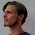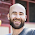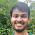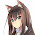## Saturday, January 28, 2012

### Lenses

Haskell's Lens type generalizes properties (i.e. accessors/mutators) found in other programming languages. For example, C# provides language support to treat properties as ordinary variables:
```class Point {
public double x { get; set; }
public double y { get; set; } }

class Circle {
public Point  center { get; set; }
public double radius { get; set; } }

public void goRight(ref Circle c) { c.center.x += 10; }
```
The initial naive implementation of the same Haskell data types and accessor/mutator functions would be:
```data Point = Point Double Double

getX :: Point -> Double
getX (Point a _) = a

setX :: Double -> Point -> Point
setX x' (Point _ y) = Point x' y

getY :: Point -> Double
getY (Point _ b) = b

setY :: Double -> Point -> Point
setY y' (Point x _) = Point x y'

data Circle = Circle Point Double

getCenter :: Circle -> Point
getCenter (Circle p _) = p

setCenter :: Point -> Circle -> Circle
setCenter p' (Circle _ r) = Circle p' r

getRadius (Circle _ r) = r

setRadius :: Double -> Circle -> Circle
setRadius r' (Circle p _) = Circle p r'
```
Hmm, this seems like a lame way to declare accessor and mutator functions. You could imagine that this would get out of hand pretty quickly if our record had lots of fields:
`getField8 (Record _ _ _ _ _ _ _ x _ _) = x`
Everybody recognized that this introduced a ridiculous amount of boilerplate, so Haskell's language designers added some syntactic sugar to automatically derive accessor and mutator functions for complicated records:
```data Point  = Point  { x :: Double, y :: Double }
deriving (Show)
data Circle = Circle { center :: Point, radius :: Double }
deriving (Show)
```
Using this syntactic sugar, you can modify individual fields using their names:
```set42Radius c = c { radius = 42.0 }
```
... and you can use the field names as accessor functions:
```>>> radius (Circle (Point 3.0 4.0) 5.0)
5.0
```
But what if we wanted to implement the Haskell equivalent of the goRight function I defined in C#? Using record syntax, we would write:
```goRight c = c { center = (\p -> p { x = x p + 10 }) (center c) }
```
Uuuuuuuggggly! As you can see, Haskell's record system is not "first-class", which is a polite way of saying it's terrible. For example, you can't use record syntax to create point-free functions:
```setX10 :: Point -> Point
setX10 p = p { x = 10 } -- valid
setX10'  =   { x = 10 } -- invalid!```
However, we can work around this by writing get and set functions, in the spirit of Java:
```getX :: Point -> Double
getX = x

setX :: Double -> Point -> Point
setX x' p = p { x = x' }```
... but this forces us to write the Haskell equivalent of ugly Java code:
```Java   : p.setX(p.getX() + 10)
Haskell: setX (getX p + 10) p
```
Because of these limitations, Haskell's record syntax crashes and burns on more complicated record hierarchies, becoming unmaintainable in a hurry.

#### Lenses

However, Haskell doesn't require language support for implementing properties! After all, a property consists of a getter and setter, so we can package them both up into a single data type, which we call a Lens instead of a property.
```                  getter,    setter
type Lens a b = ( a -> b , b -> a -> a )
```
So now we can write more general get and set functions:
```getL :: Lens a b -> a -> b
getL (g, _) = g
-- or  getL = fst

setL :: Lens a b -> b -> a -> a
setL (_, s) = s
-- or  setL = snd

-- Apply a function to the field
-- I consider modL more fundamental than setL
modL :: Lens a b -> (b -> b) -> a -> a
modL l f a = setL l (f (getL l a)) a

x' :: Lens Point Double
x' = (getX, setX)

>>> getL x' (Point 3.0 4.0)
3.0
>>> setL x' 10.0 (Point 3.0 4.0)
Point {x = 10.0, y = 4.0}
```
We are slightly improving on Java notationally, but still way behind C#. However, we're just getting started. First off, we can take advantage of Haskell's syntactic support for operators, which are just infix functions:
```(^.) :: a -> Lens a b -> b
a ^. p     = getL p a
-- or (^.) = flip getL

(^=) :: Lens a b -> b -> a -> a
(p ^= b) a = setL p b a
-- or (^=) = setL

>>> (Point 3.0 4.0) ^. x'
3.0
>>> (x' ^= 10.0) (Point 3.0 4.0)
Point {x = 10.0, y = 4.0}```
That looks better, but it still resembles a functional style, especially the setter. What we really want is something stateful, in the spirit of imperative programming. Fortunately for us, we can use the State monad to implement an imperative programming style:
```-- stateful version of (^=)
(^:=) :: Lens a b -> b -> State a ()
p ^:= b = do
a <- get -- read the current state
let a' = setL p b a
put a'   -- set the current state
-- or p ^:= b = modify (setL p b)

access :: Lens a b -> State a b
access p = do
a <- get -- read the current state
let b = getL p a
return b -- return the value
-- or access p = gets (getL p)```
Now we can write all our favorite imperative functions using the above two primitives:
```-- modify field 'p' using function f
(%=) :: Lens a b -> (b -> b') -> State a ()
p %= f = do
b <- access p
p ^:= f b

p += x = p %= (+ x)
p *= x = p %= (* x)

y' :: Lens Point Double
y' = (getY, setY)

goUp :: State Point ()
goUp = y' += 7.0

>>> :type (execState goUp)
execState goUp :: Point -> Point
>>> execState goUp (Point 3.0 4.0)
Point {x = 3.0, y = 11.0}
```
Fortunately for you, Edward Kmett already wrote all these functions for you and provides them in the data-lens package. The only difference is that he factored the Lens type into a more efficient and elegant form and some of the operators use different symbols.

#### Categories

So if all we could do was ape C# syntax I wouldn't be writing this blog post, so it's time to dig a little deeper.

Haskell's greatest strength stems from the fact that it derives all of its design patterns from mathematics, specifically category theory. Haskell borrows the Category design pattern from category theory, which is defined as anything that implements composition and identity:
```class Category c where
(.) :: c y z -> c x y -> c x z
id :: c x x
```
Obviously functions form a Category:
```instance Category (->) where
(f . g) x = f (g x)
id x = x
```
However, lenses form a Category, too!
```instance Category Lens where
... -- exercise for the reader!
```
Any Category instance must satisfy three laws (know as the Category Laws):
• Left Identity: id . f = f
• Right Identity: f . id = f
• Associativity: (f . g) . h = f . (g . h)
Other than that, anything goes, which is why categories appear in so many unexpected places. Since Lenses are categories we can compose them, which results in a new Lens which composes their their accessor and mutator functions. In other words:
```getL id = id
getL (lens1 . lens2) = getL lens1 . getL lens2
-- i.e. getL defines a Functor

modL l id = id
modL l (f . g) = modL l f . modL l g
-- (modL l) defines a Functor, too
```
In fact, you can use the above two definitions to derive the Category instance for Lens.

This leads us to Haskell's verbatim translation of our original goRight function:
```goRight = (x' . center') += 10

>>> :type x'
x' :: Lens Point Double
>>> :type center'
center' :: Lens Circle Point
>>> :type (x' . center')
x' . center' :: Lens Circle Double
>>> let c = Circle (Point 3.0 4.0) 5.0
>>> c ^. (x' . center)
3.0
>>> c ^. center' ^. x'
3.0
>>> execState goRight c
Circle {center = Point {x = 13.0, y = 4.0}, radius = 5.0}```
The Category class enforces seamless composition because of the Category laws. For example, when we compose two lenses, we can't "uncompose" the result because it is absolutely indistinguishable from the equivalent hand-written lens. This is what "seamless" means: the "seam" between the two lenses completely vanishes when we compose them. The Category design pattern guarantees leak-proof abstraction.

• Haskell's lenses behave like "first-class l-values": You can pass lenses as arguments to functions, modify them or compose them and despite all of this you can still assign to the resulting expression. You don't need to read a long language specification to know whether or not a certain expression qualifies as something assignable. If it's a Lens, it's assignable, period.
• Haskell lenses are implemented using ordinary, pure functions. Haskell language designers never built Lens into the language, which means that if you disagree with its implementation, you can implement your own version! In fact, Hackage lists several lens packages, although fclabels and data-lens are in my opinion the two best implementations at the moment.
• Lenses are easier to combine. You don't have to write a new getter and setter to combine two lenses. Just compose them and you're done. Use all that time you save to do whatever it is Haskell programmers do with all the free time they supposedly have.
Lenses still require boilerplate lens definitions, but many solutions exist to this problem. Most lens packages provide Template Haskell macros to automatically derive lenses for records. That means that the final idiomatic Haskell equivalent to the original C# code would be:
```data Point = Point { _x :: Double, _y :: Double }
data Circle = Circle { _center :: Point, _radius :: Double }
\$( makeLenses [''Point, ''Circle] ) -- <- Template Haskell

goRight = (x . center) += 10
```
Template Haskell is poorly thought out in my opinion, but there are some ideas to replace it with a much more powerful template system that can be statically checked. That makes a very interesting topic in its own right, which I'll visit in a later post.

1.s/Property a b /Lens a b/

2.access :: Lens a b -> State a b
access = do
a <- get -- read the current state
let b = getL p a
return b -- return the value

should include the parameter `p`, like so:

access :: Lens a b -> State a b
access p = do
a <- get -- read the current state
let b = getL p a
return b -- return the value

3.Also,

>>> execState goUp (Point 3.0 4.0)
Point {x = 10.0, y = 4.0}

seems wrong. It should be, if I'm correct,

>>> execState goUp (Point 3.0 4.0)
Point {x = 3.0, y = 11.0}

1.Thank you so much. Great catches! I believe I fixed all of them. I really appreciate it.

2.Don't mention it! :)

Thanks for the easily followed "tutorial". Me likes!

As a suggestion, I would like to see a guide about how to actually use fclabels and/or data-lens, since the hackage:d packages have no "how to" material whatsoever (although your material here goes a long way). I could even help, if there's anything to help with. I do so like this concept about `Lens`:es. :)

3.Yeah, I will, although I want to wait for the more recent lens discussions on reddit to settle down because it looks like more powerful versions are on the way.

4.4.This comment has been removed by the author.

1.This comment has been removed by the author.

5.OK, I obviously can't press the right buttons; hence the two removals above :) What I wanted to say was this:

I am referencing this article on my amateur Haskell blog: http://monoid.se/lenses/. If you find anything objectionable, write me a line.

6.Great article! As a newbie to haskell this has been very enlightening. Now I'm going to rummage through your blog for more :-)

1.Thanks! I'll try to write some more introductory posts in the near future, too.

7.A very nice introduction to lenses, thank you! I sure as hell approve your slam on the record syntax. I can't wait for something to be at last done about it in the language specs. Btw do are there any news on when it's going to happen?

1.So I think that the general consensus is that the new `lens` package has the right type for lenses. The reason people really like the new lens type is:

a) You don't even have to import the package to define lenses
b) It's very very elegant and "self-evident" (i.e. there is exactly one right way to implement it)
c) It supports polymorphic update
d) It supports write-only and read-only lenses

Point (a) is very important, because if we define the new record syntax to use lenses you don't want the language definition to dependent on a particular package's data type. The new formulation of a lens requires only `Functor`s, so it is very easy to define a language specification that can automatically derive lenses for an arbitrary data type, even one with multiple constructors.

However, although the new lens type is essentially nailed down at this point, people are still debating over what the standard library of lens operators should look like. Edward has done a lot of work with his `lens` package showing the full range of power of the new lenses, but it is a very large and unapproachable package and I think people are waiting for him to distill out a smaller Haskell98 core package with few dependencies before they add lenses to the language.

8.Thanks for the article, it has been nicely written.

A small typo: s/x/x'

in

>>> (Point 3.0 4.0) ^. x
3.0
>>> (x ^= 10.0) (Point 3.0 4.0)
Point {x = 10.0, y = 4.0}

1.Thank you! I fixed it.